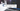# Add two numbers(reverse order) link list Problem - Leet Code Solution

July 23, 2019## Problem Statement

You are given two non-empty linked lists representing two non-negative integers. The digits are stored in reverse order and each of their nodes contain a single digit. Add the two numbers and return it as a linked list.

You may assume the two numbers do not contain any leading zero, except the number 0 itself.

``````Example:

Input: (2 -> 4 -> 3) + (5 -> 6 -> 4)
Output: 7 -> 0 -> 8
Explanation: 342 + 465 = 807.``````## Solution

``````private static ListNode addTwoNumbersHelper(ListNode l1, ListNode l2, int carry) {
if (l1 == null && l2 == null && carry == 0) {
return null;
}

int sum = carry;
if (l1 != null) { sum += l1.val; }
if (l2 != null) { sum += l2.val; }

if (sum > 9) {
sum = sum - 10;
carry = 1;
}
else {
carry = 0;
}

ListNode newNode = new ListNode(sum);
newNode.next = addTwoNumbersHelper(l1 != null ? l1.next : null, l2 != null ? l2.next : null, carry);
return newNode;
}
public static ListNode addTwoNumbers(ListNode l1, ListNode l2) {
}``````

## Explanation

This problem is rather simple. Since, the number is represented as link list in reverse order. i.e. the first node represent the last digit of a number. So, you can just started adding number as you traverse the two link list.